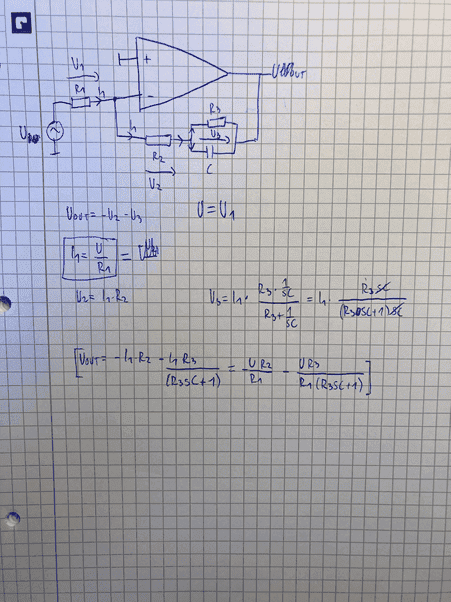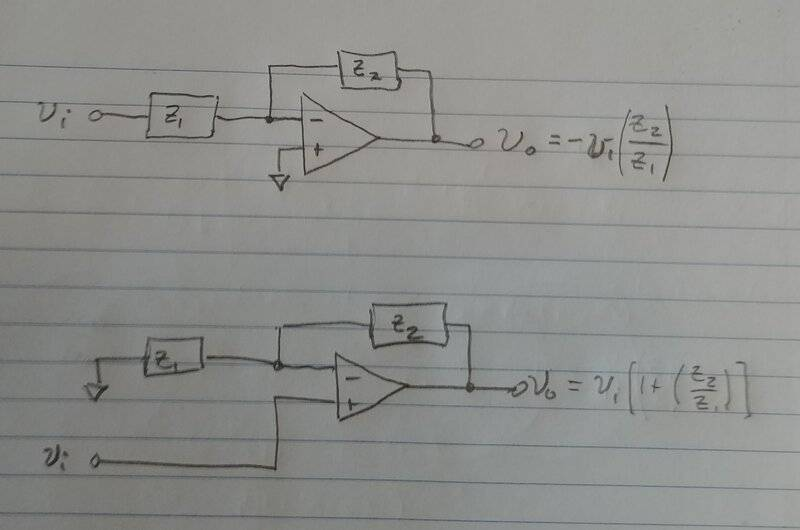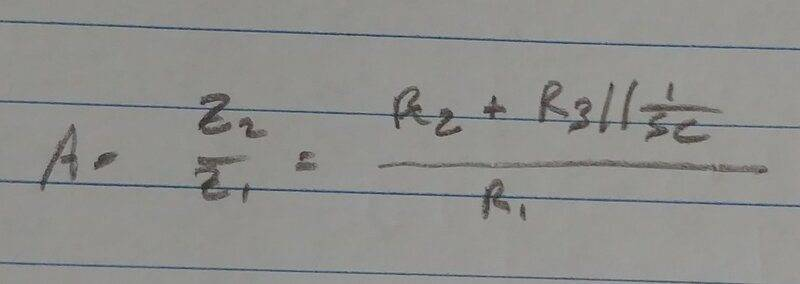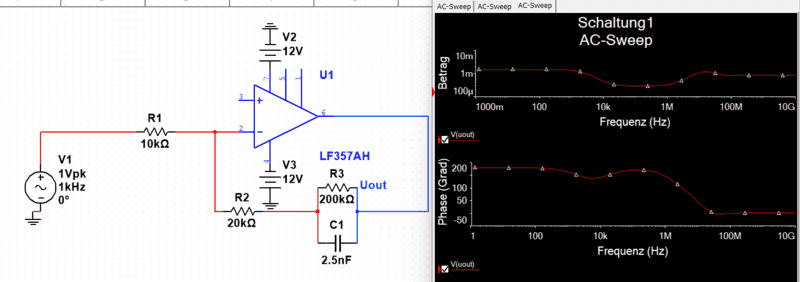# Op amp circuit -- Did I calculate the output voltage correctly?

• Engineering
• altruan23
In summary, LvW, you should connect the + input to your op-amp to ground. You should then find the transfer function in the factored pole-zero form and substitute the complex impedance for Z1 & Z2. You can then draw the bode plot.

#### altruan23

Homework Statement
find the output voltage in op amp circuit
Relevant Equations
voltage divider, ohms lawcan someone check, if i calculated the output voltage correct? i used virtual ground because V+-input of the op amp is 0 --> V- input is then also 0.

Yes, looks good to me. In my work, I would keep simplifying to get a factored pole-zero form like this:
$$V_o = - U \left [\frac{R_2+R_3}{R_1} \right ] \left [\frac{(1+s(R_2 \parallel R_3)C)}{(1+sR_3C)} \right ]$$
This canonical form allows quick insight into the nature of the circuit. Then the DC gain, poles, and zeros are readily apparent.

PS: ## a \parallel b = \frac{ab}{a+b} ##

edit: Oops! Left out the - sign.

Last edited:
•altruan23
thanks again for the help!

•DaveE
It's great to work this circuit out from basic principles, like you did. But, in the future, you'll want to use a shortcut by memorizing the answer to the simple op-amp configurations below. It will save you a lot of effort later.Then you can just substitute the complex impedance for Z1 & Z2. This can initially be done just by inspection. My analysis started, with nearly zero effort, like this:The rest is just a bunch of algebra to make it look the way I wanted (also noticing I'd left out the minus sign, lol).

•berkeman and altruan23
wow, really nice way to solve circuits like this. thanks , i will try to use it in the future.

Hello I have one more question. How can I draw the Bode-diagramm?
I simulated in MultiSim it schould probably look like this: Yeah i forgot also to give a logarithmic scale on the upper diagram on Y. On the lower diagram it schould be the phase.First, you need to connect the + input to your op-amp, to ground, I would suppose.

Drawing bode plots is a bit complicated to explain in a reply like this, but there are several good references on the web if you search for "how to draw a bode plot". You'll want to start by finding the transfer function (gain) in the factored pole-zero form

•altruan23
I am a bit surprised about the magnitude diagram - magnitude values in the mV range?
What did you display in this diagram? Vout/Vin ?
More than that - which opamp model did you use? Ideal or real?

Here is a really good review of Bode Plots. Look at section 8.1 "review of bode plots". Most of this book will be too advanced for you, and probably not relevant to what you are learning (yet). But this chapter starts really well. It's the whole treatment so you'll probably not finish the whole section. For now you'll want to ignore all of the stuff about quadratic roots and such.

•altruan23
alright I am going to have a look at this book. thanks!

Altruan - when you are interested in some help, why didn`t you answer my questions in my post#8?
You have shown a diagram which looks strange - but you did not state what you have shown.

Hello LvW. I am sorry that you were waiting for my reply, i just forgot so that is my mistake. Yeah the diagram is wrong, because i forgot to give a ground to the + terminal of the op amp. Thank you anyways for your help and that you cared and again sorry that i didnt answer your question.

OK - no problem Such things can happen ...if you have some specific questions - do not hesitate to ask again.